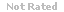Loading... Please wait...

(TCOs 1 and 2) In Chapter 9, we learned the basics of categorical deductive logic. It is important to remember that categorical arguments

Price:
\$8.99

Product Description

1. (TCOs 1 and 2) In Chapter 9, we learned the basics of categorical deductive logic. It is important to remember that categorical arguments have a specific structure and purpose. Consider the following question:
Categorical deductive logic has a long history of use and development since the time of ancient Greece. All categorical deductive arguments are considered:
2.
(TCO 4) Chapter 9, we learned the basics of categorical deductive logic. It is important to remember that categorical arguments have a specific structure and purpose. Consider the following question:
Each of the four standard-form categorical claims in categorical logic can be displayed graphically in a standard way using what logical tool?
3.
(TCOs 1 and 3) Chapter 9, we learned the basics of categorical deductive logic. It is important to remember that categorical arguments have a specific structure and purpose.
Categorical syllogisms must have two premises and three terms: the major term, the minor term, and the middle term. Consider the following example:
All cats are mammals.
Some mammals are domesticated animals.
Therefore, some cats are domesticated animals.
In this categorical syllogism, the middle term, the term in both premises but not in the conclusion, is:
4.
(TCOs 1 and 2) Chapter 9, we learned the basics of categorical deductive logic. It is important to remember that categorical arguments have a specific structure and purpose. Consider the following question:
Standard-form categorical claims require that claims in other forms be translated so that the four standard-form claims contain which two kinds of terms?
5.
(TCOs 3 and 4) In Chapter 8, we learned the basics of truth-functional logic. It is important to remember that the purpose of truth-functional logic is to determine which arguments are valid and which are invalid, and common invalid forms are known as truth-functional fallacies. Consider the following question.
Which of the following is a well-known truth-functional fallacy?

Customers Who Viewed This Product Also Viewed

• \$8.99
• \$8.99
• \$19.99
• \$8.99
• \$8.99

• \$8.99